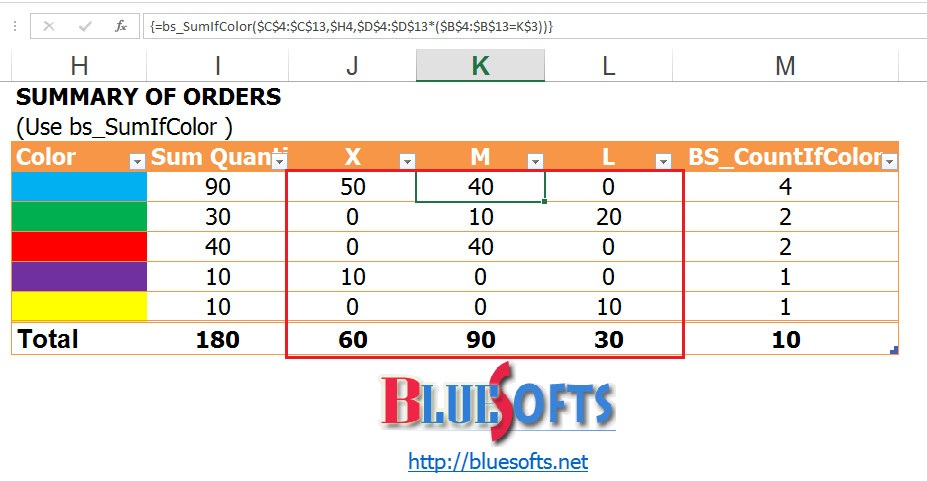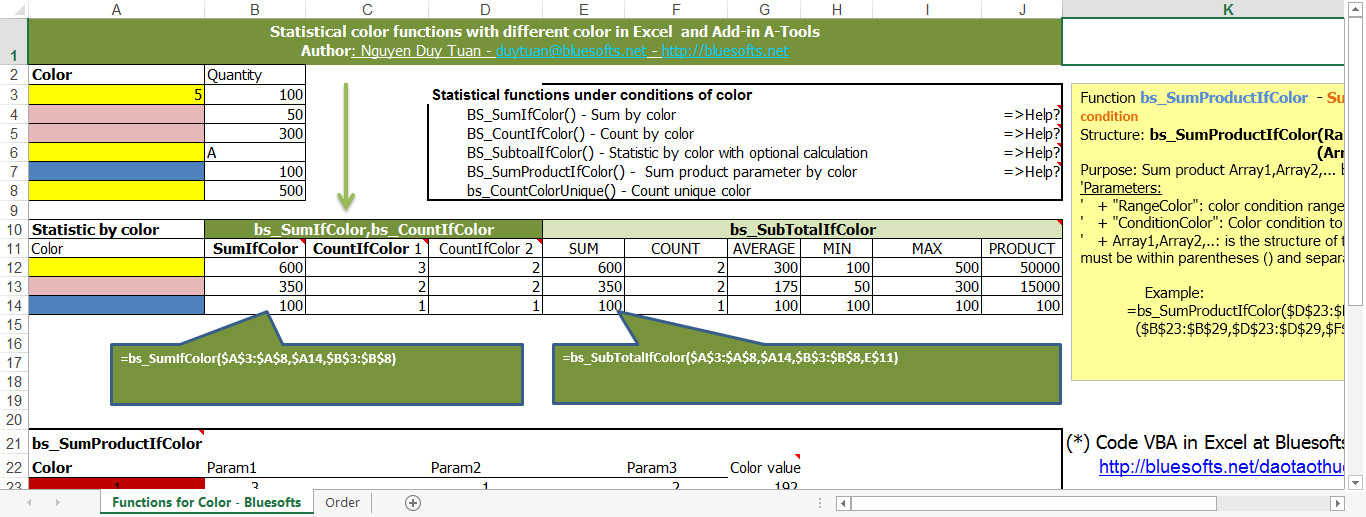You are here

# Query, Share and Manage Excel workbooks on LAN, Internet

## Statistical color functions with different color in Excel and Add-in A-Tools

I would like to send you video and article introduce how to do statistic on color in Excel and Add-in A-Tools. In this video, I will guid you how to use BS_SumIfColor, BS_CountIfColor function to total and count the color conditions and other conditions combined with an array formula. This is a special solution, flexibility with the breakthrough technology of the formula in Excel and Add-in A-Tools.

Here is the extended functions of the Add-in A-Tools consists of a lot of content related to color to support users.Suppose a company have to receive the orders from customers in garment contains information about ID, order, size, color, quantity, unit price, amount. Every day, employees enter information form to fill out the table.

So, how manager can statistically to calculate order quantity for each color.

To do that, you set up "The orders" report by color, we need to calculate the number of colors.

Guide

1.  BS_SumIfColor Formula

Place the cursor in the box to be calculated, on the A-Tools menu, select "Expansion Function", then select content "BS_SumIfColor"the table settings will show us the following information:

+ "Range Color": Conditional color range, we press F4 to absolute.+ "Condition Color": The cell contains color conditions that we want to sum.+ "Range Calc": Range calculation, we press F4 to absolute.

Then we click "OK" to complete the installation and display the results.We've done the sums for each color, the results are displayed as follows(Or we can type the formula directly if we have known the structure of the BS_SumIfColor formula)

2. BS_CountIfColor Formula

Place the cursor in the box to be calculated, on the A-Tools menu, select "Expansion Function", then select content "BS_CountIfColor”, then fill out setting tables as BS_SumIfColor formula. Inside:

+ "Range Color": We choose the color as the conditions.

+ "Condition Color": The conditions of color, we only need color box statistics.

+ "Range Calc": Region to count, we highlight the number of colors.

Note for the "Range Color" and "Range Calc", we press F4 to absolute.

Then we press the "OK" button to display the results.Results are shown as follows:(Or we can type the formula directly if we have the structure of the jaw BS_CountIfColor)

3. Calculate the total under many conditions

We do the same operations as a function BS_SumIfColor, after perfecting the formula, in the calculation, we add the condition by adding a logical expression * (the size = cell size) as guidelines:Once done, we press Ctrl + Shift + Enter to display the results.

Drag the mouse sideways and downwards to copy the formula to other cells of data.Formula A-Tools flexible when we changed the color is selected, the formula will update the value for us, when we agree click to select.You can refer to the color system of existing functions in Excel.